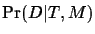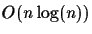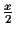Next: Definitions Up: Introduction Previous: Distance Matrix Methods

#### Maximum Likelihood

The maximum likelihood (ML) approach chooses the tree which maximizes the probability that the observed data would have occurred. We have a model M and the data D. The likelihood of a tree T is the probability of the data D given the tree T and the model M:(2)

When the data are fixed and the tree varies, then the values of allfor all T need not add to one. These are called likelihoods instead of probabilities. One candidate for the best tree is the tree that maximizes the likelihood. The strategy is to search over all trees, and for each topology T to find the lengths of the edges that maximize the likelihood P(D|T). The topology and the assignment of edge lengths that give the overall maximum of this likelihood is the desired tree.
We are interested in tree construction methods that only need the raw, unaligned sequences as input and do not require any further information such as an MSA. In this extended abstract, we present an algorithm that runs intime and produces a tree with optimal score, if the error of each distance is not greater than, where x is the shortest edge in the tree. In the following sections we explain our tree scoring function. We then introduce the main ideas and algorithm. Finally, we show how the error influences the results and present experimental results.Next: Definitions Up: Introduction Previous: Distance Matrix Methods
Chantal Korostensky
1999-07-14